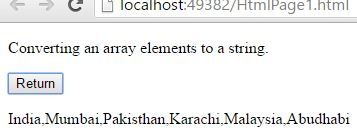# Array to String in JavaScript

#### Array to String

Array to string means converting an array into a string. In JavaScript the conversion of Array to String possible with the help of `toString()` method. The `toString()` method does not have any parameters.

NOTE: In string references also `toString()` method is used, in that string references `toString()` method return the value of a string.

In JavaScript Arrays, by using `toString()` method we can convert an array into a string.

EXAMPLE: Converting an array which contains elements to a string.

```<p>Converting an array elements to a string.</p>
<input type="button" onclick="myValue()" value="Return"/>
<p id="myId"></p>

<script>
function myValue() {
var a = ["India", "Mumbai", "Pakisthan", "Karachi", "Malaysia", "Abudhabi"];
var b = a.toString();
document.getElementById("myId").innerHTML = b;
}
</script>
```

In the above code snippet we have given `Id` as "`myId`"to the second `<p>` element in the HTML code. There is a function myValue() in the`<script>`block which is connected to the onclick of the HTML button and array with value "["India", "Mumbai", "Pakisthan", "Karachi", "Malaysia", "Abudhabi"]" to the variable `a`. We need to convert an array into a string, for that we are using `toString()` method, this method converts the elements of an array into a string and returns the each element in the output seperated by comma (,) operator. Onclick of the button "Return" in the HTML code fires the function myValue() in the `<script>` block, at the same time `toString()` method converts the elements in an array to string and returns as output.

OUTPUTIn the above output we can notice that the each element is seperated by comma (,) operator.

EXAMPLE: Converting an array which contains numbers to a string.

```<p>Converting an array numbers to a string.</p>
<input type="button" onclick="myValue()" value="Return"/>
<p id="myId"></p>

<script>
function myValue() {
var a = [52, 36, 85, 24, 69, 47, 32, 3, 4, 8];
var b = a.toString();
document.getElementById("myId").innerHTML = b;
}
</script>
```

Converting array numbers into a string

Onclick of the button "Return" in the HTML code fires the function myValue() in the `<script>` block, at the same time `toString()` method converts the numbers in an array to string and returns as output.

OUTPUTViews: 3223 | Post Order: 160